### Fractions and Decimals - Revision Notes

CBSE Class–VII Subject Mathematics
Revision Notes
Chapter-2
Fractions and Decimals
• We have learnt about fractions and decimals along with the operations of addition and subtraction on them, in the earlier class.
• We now study the operations of multiplication and division on fractions as well as on decimals.
• We have learnt how to multiply fractions. Two fractions are multiplied by multiplying their numerators and denominators seprately and writing the product as product of numerators by product of denominators. For example,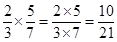.
• A fraction acts as an operator ‘of ’. For example,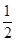of 2 is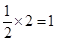.
(a) The product of two proper fractions is less than each of the fractions that are multiplied.
(b) The product of a proper and an improper fraction is less than the improper fraction and greater than the proper fraction.
(c) The product of two improper fractions is greater than the two fractions.
• A reciprocal of a fraction is obtained by inverting it upside down. We have seen how to divide two fractions.
(a) While dividing a whole number by a fraction, we multiply the whole number with the reciprocal of that fraction. For example,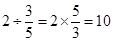.
(b) While dividing a fraction by a whole number we multiply the fraction by the reciprocal of the whole number. For example,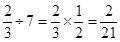.
(c) While dividing one fraction by another fraction, we multiply the first fraction by the reciprocal of the other. So,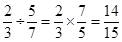.
• We also leant how to multiply two decimal numbers. While multiplying two decimal numbers, first multiply them as whole numbers. Count the number of digits to the right of the decimal point in both the decimal numbers. Add the number of digits counted. Put the decimal point in the product by counting the digits from its rightmost place. The count should be the sum obtained earlier. For example,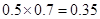.
• To multiply a decimal number by 10, 100 or 1000, we move the decimal point in the number to the right by as many places as there are zeros over 1. Thus,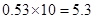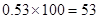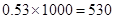.

• We have seen how to divide decimal numbers.
(a)To divide a decimal number by a whole number, we first divide them as whole numbers. Then place the decimal point in the quotient as in the decimal number. For example,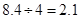.

Note that here we consider only those divisions in which the remainder is zero.
(b)To divide a decimal number by 10, 100 or 1000, shift the digits in the decimal number to the left by as many places as there are zeros over 1, to get the quotient. So,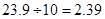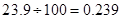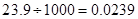.
(c) While dividing two decimal numbers, first shift the decimal point to the right by equal number of places in both, to convert the divisor to a whole number. Then divide. Thus,